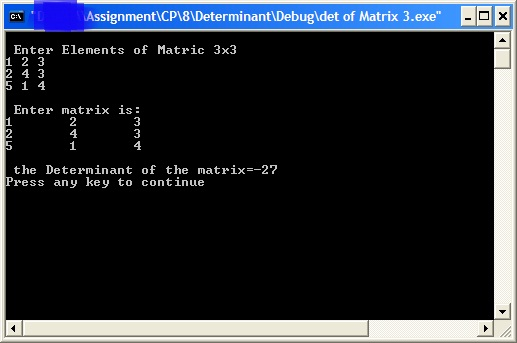## Wap in c to find determinant of matrix order 3x3 , C/C++ Programming

Assignment Help:

WAP in C to find determinant of matrix order 3x3

#include

#include

void main()

{

int i, j,cal;

int det;

printf("\n Enter Elements of Matric 3x3\n");

for (i=0; i<3; i++)

{

for(j=0;j<3; j++)

{

scanf("%d",&det[i][j]);

}

}

printf("\n Enter matrix is:\n");

for (i=0;i<3;i++)

{

for(j=0;j<3; j++)

printf("%d\t",det[i][j]);

printf("\n");

}

// Determinants of the matrix

cal=(det)*(cal=(det*det)-(det*det))-det*((det*det)-(det*det))+det*((det*det)-(det*det));

printf("\n the Determinant of the matrix=%d\n",cal);

}

OUTPUT#### Write a program that finds the minimum total number of she., Write a progra...

Write a program that finds the minimum total number of shelv, C/C++ Programming

#### Enumeration types, Write a simple program in C++ to investigate the safety ...

Write a simple program in C++ to investigate the safety of its enumeration types. Include at least 10 different operations on enumeration types that are incorrect/unsafe things to

#### File handling, one programming exampale

one programming exampale

program

#### Software crisis, software crisis definition

software crisis definition

#### Array of structure, how to define a array of structure

how to define a array of structure

#### develop a calculator in masm, Part 1 Assignment:  develop a calculator...

Part 1 Assignment:  develop a calculator in MASM. Text chapters covered:  1 through 4, 5.4, 5.5, 6.3, 7.4 You will develop a "calculator" algorithm in MASM using reverse-

#### Super ASCII string cost, string will contain only lower case alphabet& the ...

string will contain only lower case alphabet& the ascii value starts from 1-26,(ie,a=1&z=26).it perform the operation like the following costs:add=2units,replace=1unit,delete=3unit

#### Write a program to illustrate passing structure to function, Write a Progra...

Write a Program to illustrate passing structure to function? # include struct customer { int id; char name; }; void func_struct(struct customer); main() { struct c

#### Structures, how to define the structures

how to define the structures